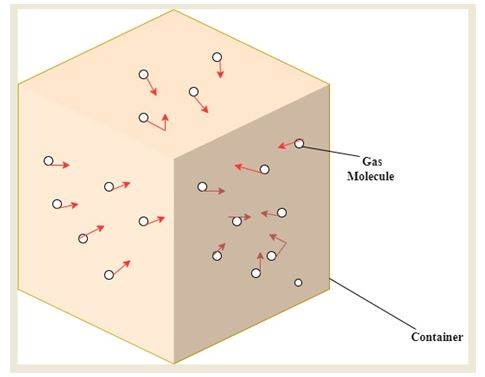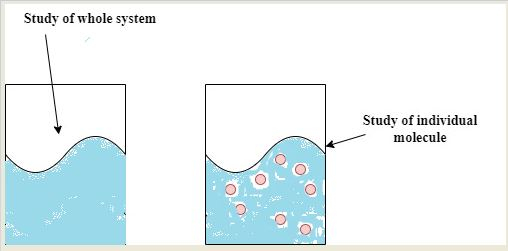# Statistical Mechanics

## Introduction

Statistical mechanics is widely considered to be a major pillar of modern physics. This topic is useful in the study of a physical system with multiple degrees of freedom. This particular approach is considered probability theory, statistical methods and microscopic physical laws. The term statistics mechanics was first postulated by J. Willard Gibbs in 1884. This mechanics is highly advantageous in obtaining the exact approach that is needed to be taken to connect the thermodynamic quantities to microscopic behaviour.

## Statistical Mechanics: Definition

This is the sub-division of the theoretical physics that studies the sausage of the probability theory and it is also the mean behaviour of a mechanical system in which the condition of the system is particularly uncertain. The most common application of this field of study is in the thermodynamic achievement of large scale systems. The statistical mechanics itself is subdivided into two sub strata namely the statistical thermodynamics and the statistical mechanics.

This mechanics is particularly helpful to give a details outline of the particular methods that are associated with connecting the thermodynamic quantities to the microscopic behaviour. The statistical nature of this mechanics also opens a horizon for extending the laws of thermodynamics with a certain quantifiable amount of freedom.

## Statistics Mechanics: Principles

Two types of mechanics govern the functionality of physics, one, classical mechanics and secondly quantum mechanics. According to Cowan (2021), there is however a standard mathematical approach associated with it that determines their particular properties. Although it is to be noted that there is a certain extent of disconnection between the daily experience and the law that is theoretically outlined because there is no necessity to be found to study these laws at a microscopic level to study the simultaneous position and velocity of every single molecule it is particularly difficult carrying this study at a human scale.

Statistical mechanics helps in filling up this disconnection that is observed between practical experience and the laws of thoroughly incomplete knowledge. It is done by the addition of some uncertainty about the condition of the system.

However, with all the technicalities considered ordinary mechanics only considers the behaviour of a single state. The statistical mechanics on the other hand introduces the statistical ensemble, which is a large collection of dependent and yet virtual copies of the system in multiple states.## Statistical Thermodynamics

The statistical thermodynamics is useful in the derivation of the classical thermodynamics of multiple objects that comes in terms of the properties of the particles present in the objects. As opined by Feynnman (2018), it also helps in establishing a relationship between the interactions between the particles. In order to define in simple terms, it can be noted that the statistical thermodynamics provides a connection between the macroscopic aspects of the materials that come in a state of a thermodynamic equilibrium. It also notes the microscopic behaviour and the motion occurring among the constituent particles of the material.

On the other hand, the statistical mechanics involves dynamics and it is solely focused on the equilibrium of statistics.## Non-Equilibrium Thermodynamics

There are multiple numbers of physical phenomena associated with an interest that involves quasi-thermodynamic processes of the state of equilibrium. Some of the examples are outlined as follows. According to Prigogine (2017), that is the transport of heat professed by the internal motions existing within a material and this motion is mostly driven by the notable imbalance that occurs in the temperature.

The currents that are transported by the relative motion of the charges noted in a conductor are thoroughly modulated by the imbalance that occurs in the state of voltage. The spontaneous chemical reaction that occurs in between the material is governed by the lowering of the free energy. In general, it can be defined that the nonequilibrium condition is an irreversible process. There are several factors associated with it, for example, friction, quantum decoherence and dissipation. The nonequilibrium thermodynamics also governs the systems that are motivated by multiple external forces.

## Conclusion

The statistical mechanics is considered one of the major branches of study of the theoretical aspect of modern physics. This study is very crucial in studying the physical system with multiple degrees of independence associated with it. The approach for the study is commonly based on multiple theories including the probability theory, statistical methods and the associated physical laws at the microscopic level. There is a statistical method associated with this machine that helps in the extension of the laws of thermodynamics with limited degrees of freedom.

## FAQs

Q.1. What is statistical mechanics?

Ans. The statistical mechanics is a study associated with the branch h of theoretical physics. The statistical mechanics is mostly beneficial in using various mechanics that are crucial to providing exact methods that connect the thermodynamic quantities to a very minuscule, microscopic level of study.

Q.2. What is the relation between thermodynamics and statistical mechanics?

Ans. The statistical mechanics is closely related to the aspect of thermodynamics in a manner that it helps in the derivation of the oldest laws and principles of thermodynamics. It is so done by the application of statistical methods regarding atoms and molecules. For a certain model of material, this mechanism even permits an individual to assess the materials constant.

Q.3. What assumptions are associated with statistical mechanics?

Ans. The statistical mechanics regarding the fundamental assumption states that with the continuation of time, an isolated system in a provided microstate is likely to stay in the microstate, in such case the energy is divided equally.

Q.4. Who coined the term statistical mechanics?

Ans. The term statistical mechanics was first given by J. Willard Gibbs; he was an American mathematical physicist. He has given this term back in 1884. He had outlined the principles in his book elementary principle of static mechanism.